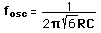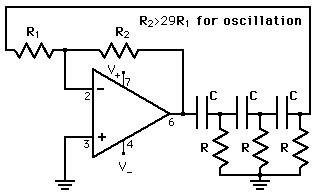# Phase-Shift Oscillator

 The phase shift oscillator produces positive feedback by using an inverting amplifier and adding another 180° of phase shift with the three high-pass filter circuits. It produces this 180° phase shift for only one frequency:at which the feedback fraction is B=1/29.For component values

Capacitor C = mF
Resistor R = KW

the frequency is f = kHz = MHz = x10^ Hz

Calculation notes: If component values are changed, the new frequency will be calculated. If a frequency is entered, then you must click on the active component name of the component you wish to calculate to force the frequency to match the entered value.

The frequency expression and the 1/29 feedback factor are derived in Appendix B of Floyd, Electronic Devices. Long, messy expressions!

 Other Oscillators
Index

Electronics concepts

Op-amp concepts

 HyperPhysics*****Electricity and magnetism R Nave
Go Back